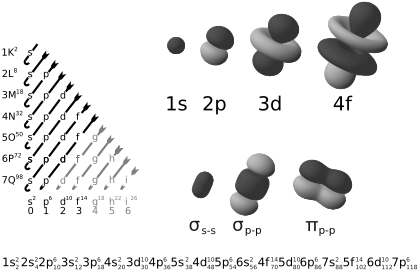# Atomic orbital facts for kids

Kids Encyclopedia FactsThe diagram to the left shows the orbitals in order of increasing energy. To the right are examples of orbital shapes.

Atomic orbitals are the places surrounding the nucleus of an atom where the electrons are most likely to be at any given time. It is a mathematical function that describes the wave-like behavior of either one electron or a pair of electrons in an atom.

The word 'orbital' is used because it was thought that electrons behaved similarly to the solar system, where the nucleus is like the sun and the electrons orbit like the planets.

The number of atomic orbitals in an element is defined by the period the element is in. Electrons move between orbitals depending on how fast they are moving and how many other electrons there are.

In atomic theory and quantum mechanics, an atomic orbital is a quantum number. Each such orbital can be occupied by one or two electrons. The way orbitals are arranged is related to the electron configurations of atoms. They were derived from descriptions provided by early spectroscopists of certain alkali metal spectroscopic lines as being sharp, principal, diffuse, and fundamental.

## Images for kidsAtomic orbital Facts for Kids. Kiddle Encyclopedia.